# RS Aggarwal Class 7 Solutions Chapter 3 Decimals Ex 3B

In this chapter, we provide RS Aggarwal Solutions for Class 7 Chapter 3 Decimals Ex 3B for English medium students, Which will very helpful for every student in their exams. Students can download the latest RS Aggarwal Solutions for Class 7 Chapter 3 Decimals Ex 3B Maths pdf, free RS Aggarwal Solutions Class 7 Chapter 3 Decimals Ex 3B Maths book pdf download. Now you will get step by step solution to each question.

### RS Aggarwal Solutions for Class 7 Chapter 3 Decimals Ex 3B Download PDF

Question 1.
Solution:
Converting them into like decimals 16.00, 8.70, 0.94, 6.80 and 7.77
16.0 + 8.70 + 0.94 + 6.80 + 7.77 = 40.21Question 2.
Solution:
Converting them into like decimals 18.600, 206.370, 8.008, 26.400, 6.900
18.600 + 206.370 + 8.008 + 26.400 + 6.900 = 266.278Question 3.
Solution:
Converting them into like decimals, 63.50, 9.70, 0.80, 26.66, 12.17
63.50 + 9.70 + 0.80 + 26.66 + 12.17 = 112.83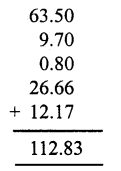Question 4.
Solution:
Converting them into like decimals 17.400, 86.390, 9.435, 8.800, 0.060
17.400 + 86.390 + 9.435 + 8.800 + 0.060 = 122.085Question 5.
Solution:
Converting them into like decimals 26.900, 19.740, 231.769, 0.048
26.900 + 19.740 + 231.769 + 0.048 = 278.457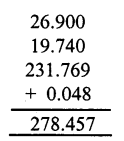Question 6.
Solution:
Converting them into like decimals 23.800, 8.940, 0.078 and 214.600
23.800 + 8.940 + 0.078 + 214.600 = 247.418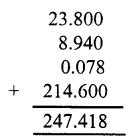Question 7.
Solution:
Converting them into like decimals.
6.606, 66.600, 666.000,0.066, 0.660
6.606 + 66.600 + 666.000 + 0.066 + 0,660 = 739.932Question 8.
Solution:
9.090, 0.909, 99.900, 9.990, 0.099
9.090 + 0.909 + 99.900 + 9.990 + 0.099 = 119.988Subtract:
Question 9.
Solution:
14.79 from 72.43
72.43 – 14.79 = 57.64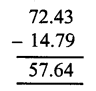Question 10.
Solution:
Converting them into like decimals, We get
36.74 and 52.60
Now 52.60 – 36.74 = 15.86Question 11.
Solution:
Converting them into like decimals, We get
13.876 and 22.000
22.000 – 13.876 = 8.124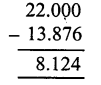Question 12.
Solution:
Converting them into like decimals, We get
15.079 and 24.160
24.160 – 15.079 = 9.081Question 13.
Solution:
Converting them into like decimals We get
0.680 and 1.007
1.007 – 0.680 = 0.327Question 14.
Solution:
Converting them into like decimals,
We get 0.4678 and 5.0500
5.0500 – 0.4678 = 4.5822Question 15.
Solution:
Converting them into like decimals,
We get 2.5307 and 8.0000
8.0 – 2.5307 = 5.4693Question 16.
Solution:
There are like decimals
9.1 – 6.732 = 2.269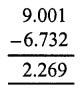Question 17.
Solution:
Converting them into like decimals,
We get 5.746 and 9.100
9.100 – 5.746 = 3.354Question 18.
Solution:
Converting into like decimals, we get,
63.59 and 92.00
Required number = 92.00 – 63.58 = 28.42Question 19.
Solution:
Converting into like decimals, we get:
8.100 and 0.813
Required number = 8.100 – 0.813 = 7.287Question 20.
Solution:
Converting them into like decimals, we get: 32.67 and 60.10
Required number = 60.10 – 32.67 = 27.43Question 21.
Solution:
Converting into like decimals, we get 74.3 and 26.87
Required number = 74.30 – 26.87 = 47.43Question 22.
Solution:
Cost of notebook = Rs. 23.75
Cost ofpencil = Rs. 2.85
Costofpen =Rs. 15.90
Total cost = Rs. 42.50
Amount gave to the shop keeper = 50 rupees
Balance amount got = Rs 50.00 – Rs 42.50 = 7.50

All Chapter RS Aggarwal Solutions For Class 7 Maths

—————————————————————————–

All Subject NCERT Exemplar Problems Solutions For Class 7

All Subject NCERT Solutions For Class 7

*************************************************

I think you got complete solutions for this chapter. If You have any queries regarding this chapter, please comment on the below section our subject teacher will answer you. We tried our best to give complete solutions so you got good marks in your exam.

If these solutions have helped you, you can also share rsaggarwalsolutions.in to your friends.